### Home > CALC > Chapter 6 > Lesson 6.2.1 > Problem6-65

6-65.
1. Solve each of the following equations for y. Homework Help ✎

1. sin y = x

2. cos−1 y = x

3. sin2 y + x2 = 1

4. 2 sin y cos y = a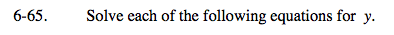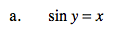y = sin−1(x)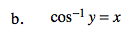See part (a).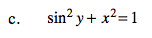Don't forget the ±.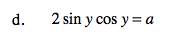2sin(y)cos(y) = sin(2y)